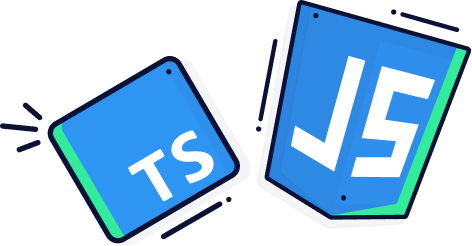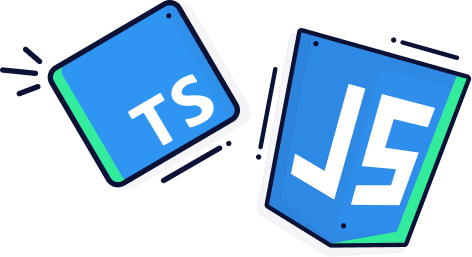Tech insights: How Haskell Helps Me to Write Safe, Expressive Code: Part 2Less noise, more data. Get the Netherlands' developer career report# How Haskell Helps Me to Write Safe, Expressive Code: Part 2

15 September 2022, by Henry Steere

Haskell is one of my favourite programming languages because it allows me to write safe code that’s easy to read and understand. Because of this and the correctness guarantees it provides, I chose to use it for Advent of Code—an advent calendar for programming problems. Here’s how I solved Day 16’s problem using Haskell’s data declarations and parsing to write an interpreter.Every day from 1 December, a new programming problem is released, increasing in difficulty as Christmas approaches. I used the challenge as programming Katas to hone my coding skills.

Day 16’s problem is interesting because:

• It requires parsing an intricately structured stream of data representing nested expressions with metadata.
• Evaluating the nested expressions is an excellent use case for an interpreter.

Haskell’s expressive data modelling and parsing features are well-suited to writing an interpreter.

## Day 16: Parsing and writing an interpreter

On day 16 of Advent of Code, Santa’s elves are sending a transmission to your submarine using the Buoyancy Interchange Transmission System, and you need to figure out the value they are transmitting to you. Data is sent as a sequence of network packets which must be parsed and interpreted as arithmetic operations. After applying all of the operations, you get the value of the transmission (your answer).

The data start out as a sequence of hexadecimal decimal characters, which must be turned into bits. The bits are then parsed into a packet format representing nested operations and values with packet metadata (a version number).

The first part of the problem requires finding the sum of the version numbers in nested packets.

The second part requires finding the value of the expression represented by the nested operations and values.

This problem requires writing an interpreter for arithmetic operations, so a good first step is deciding how these expressions will be represented by defining the data model. Once the data model is defined, the next step is parsing the input into the data model. Once the input has been parsed into the data model, we need to write an interpreter for the arithmetic expressions to compute the value. This value will be the answer to the problem.

## Expressively defining the data model

Data declarations in Haskell create struct-like types which have no functions associated with them. In this way, they are like classes in Java without any methods. For example, these two definitions create almost the same data type:

### In Java

``````public class Point {
public Point(int x, int y) {
this.x = x;
this.y = y;
}
int x;
int y;
}
``````

``````data Point Int Int
``````

However, apart from Haskell’s conciseness, they are different in an important way. Haskell data types are a combination of structs with data members called product types and enumerations called sum types. Together sum types and product types are called algebraic data types or ADTs. To declare a sum type in Haskell, you use the same data declaration as above but separate each member of the union with the pipe character ‘|’. These sum types can be exhaustively matched against, which means the compiler can prevent you from leaving out a case. This is very useful for preventing bugs.

For this problem, I defined a Haskell data type which could represent parsed packets:

``````data Packet = Literal Int Int
| Operation Int Operator deriving Show
``````

Each Packet has a version number represented as an `Int`. Packets are either `Literals` which have an `Int` value, or Operations acting on sub-packets.

``````data Operator = Sum [Packet]
| Product [Packet]
| Minimum [Packet]
| Maximum [Packet]
| Gt Packet Packet
| Lt Packet Packet
| Equal Packet Packet deriving Show
``````

There are seven possible operations:

1. `Sum` adds the values of sub-packets
2. `Product` multiplies the values of sub-packets
3. `Minimum` finds the smallest value among its sub-packets
4. `Maximum` finds the maximum value of its sub-packets
5. `Gt` returns 1 if its first argument is greater than its second argument; otherwise, 0
6. `Lt` returns 1 if its first argument is less than its second argument; otherwise, 0
7. `Equal` returns 1 if its arguments are equal; otherwise, 0

The hardest part of day 16 is parsing the input. Fortunately, Haskell is particularly suited to parsing: It has a concise and expressive syntax, useful built-in combinators, and a number of monadic combinator parsing libraries in its ecosystem.

I decided to use Parsec, the oldest monadic parsing library because it’s very expressive and fast enough. The input for Day 16 was provided as a stream of hex characters.

A059141803C000…

These characters first needed to be transformed into a sequence of bits by interpreting the hex characters as bytes. For convenience, I transformed the hex into a lazy list of characters where each character is a one or a zero:

``````bits :: GenParser Char st String
bits = (char '0' >> pure "0000")
<|> (char '1' >> pure "0001")
<|> (char '2' >> pure "0010")
<|> (char '3' >> pure "0011")
...

allBits :: GenParser Char st String
allBits = concat <\$> many1 bits
``````

The first parser translates a single hex character into a sequence of the bits representing it, and the second parser concatenates a sequence of parsed hex characters into a stream of bits.

I used helper parsers to extract integer values from the sequence of bits.

``````bit :: GenParser Char st Int
bit = (char '0' >> pure 0) <|> (char '1' >> pure 1)

intBits :: Int -> GenParser Char st Int
intBits n = bitsToInt <\$> count n bit

bitsToInt :: [Int] -> Int
bitsToInt = foldl' (\acc b -> b + 2 * acc) 0
``````

The bit function converts a ‘0’ character to an integer 0 and a ‘1’ character to 1. The intBits parser parses n bits and converts them to an integer using the `bitsToInt` function. With these helpers in place, the rest of the parsing involved reading sequences of bits from the bit stream, interpreting them as integers and deciding whether they represented parts of a literal packet or an operation packet. Here is how to parse a literal packet:

``````version :: GenParser Char st Int
version = intBits 3

middleChunk :: GenParser Char st [Int]
middleChunk = char '1' *> count 4 bit

lastChunk :: GenParser Char st [Int]
lastChunk = char '0' *> count 4 bit

literal :: GenParser Char st Packet
literal = do
v <- version
_ <- string "100"
middle <- many middleChunk
lst <- lastChunk
let result = (Literal v (bitsToInt (concat middle ++ lst)))
return \$ result
``````

A literal begins with a three-bit integer representing its version number. It then has an identifier which is the number 4 in bits or “100”. The rest of the packet is made up of chunks.

Each chunk, except the last, starts with one bit and is followed by four other bits. The last chunk in the packet contains a zero bit followed by four other bits. The value of the literal is found by concatenating the bits in the chunks, excluding the leading one or zero, and interpreting that as an integer. This algorithm is represented very directly in a monadic parser. Parsing operators is done similarly.

By representing only legal states with types, you can be sure that once you’ve parsed the data, you won’t have to write code to handle illegal states. Haskell’s type system and exhaustive matching guarantee no null values and no values that can’t be handled by structural recursion on the data model. Whenever possible, it’s a good idea to parse rather than validate.

Some other languages, like Java, don’t provide strong type guarantees, which can lead to accidental runtime exceptions. For example, when constructing a Java ZonedDateTime you need to specify the time zone with a string like “UTC”. This isn’t type-safe and will give you an exception at runtime if you get the zone’s name wrong.

Writing an interpreter for the data model

The datatype representing the packets as either literals or operations is actually a Domain Specific Language (DSL), and one of Haskell’s strengths is easily writing interpreters for DSLs.

There is a standard way to implement DSLs in Haskell. First, operations for the DSL are represented as data types (reification). Haskell’s expressive sum types and product types help define the data model. The data types are then recursively processed, and Haskell’s pattern matching provides a clear way to specify how each operation should be evaluated, case by case and exhaustively. This is called structural recursion.

Part two of Day 16 can be solved by writing an interpreter, which finds the value of a packet. Here’s how I did that.

``````packetValue :: Packet -> Int
packetValue (Literal _ v) = v
packetValue (Operation _ (Sum ps)) = sum \$ map packetValue ps
packetValue (Operation _ (Product ps)) = product \$ map packetValue ps
packetValue (Operation _ (Minimum ps)) = minimum \$ map packetValue ps
packetValue (Operation _ (Maximum ps)) = maximum \$ map packetValue ps
packetValue (Operation _ (Gt one other)) = let v1 = packetValue one
v2 = packetValue other
in if v1 > v2 then 1 else 0
packetValue (Operation _ (Lt one other)) = let v1 = packetValue one
v2 = packetValue other
in if v1 < v2 then 1 else 0
packetValue (Operation _ (Equal one other)) = let v1 = packetValue one
v2 = packetValue other
in if v1 == v2 then 1 else 0
``````

Solving Advent of Code’s Day 16 problem was a great way to use Haskell’s strong type guarantees and ensure I didn’t have any runtime exceptions.

Check out Part 1 and Part 3 of the series to see how Haskell helps me to write safe, expressive code. In Part 3, I use Haskell’s unique structural recursion on immutable trees to solve Day 18’s problem.

Henry is a Scala engineer at GrapheneDB where he uses functional programming to manage graph databases in the cloud. He loves functional programming because it makes code robust and easy to reason about.